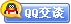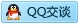##用户名 Email 自动登录 找回密码 密码 会员注册
 VIP会员，3年作业免费下 ！ 奥鹏作业，奥鹏毕业论文检测 新手作业下载教程，充值问题 没有找到答案，请在此处留言！ 2019年12月最新全国统考资料 投诉建议，加盟合作！奥鹏课程积分软件(ver:3.1)

# 12秋电子科大《C语言》在线作业3发表于 2012-10-12 10:34:07 | 显示全部楼层 |阅读模式谋学网: www.mouxue.com 主要提供奥鹏作业答案，奥鹏在线作业答案，奥鹏离线作业答案以及奥鹏毕业论文，致力打造中国最专业远程教育辅导社区。 一、单选题（共 35 道试题，共 70 分。）V 1.  当把以下四个表达式用做if语句的控制表达式时，有一个选项与其它三个选项含义不同，这个选项是( ) A. K%2 B. K%2==1 C. （K%2）！=0 D. ！K%2==1       满分：2  分 2.  以下正确的描述是( ) A. continue语句的作用是结束整个循环的执行 B. 只能在循环体内和switch语句体内使用break语句 C. 在循环体内使用break语句或continue语句的作用相同 D. 从多层循环嵌套中退出时, 只能使用goto语句       满分：2  分 3.  若有定义：int a,则对a数组的第i行j列元素地址的正确引用为( ) A. *(a+j) B. (a+i) C. *(a+j) D. a+j       满分：2  分 4.  若有如下语句: int x=3; do { printf(" %d\n",x -=2);} while(!(--x)); 则上面程序段( ) A. 输出的是 1 B. 输出的是1和-2 C. 输出的是3和0 D. 是死循环       满分：2  分 5.  C语言规定，简单变量做实参时，它和对应形参之间的数据传递方式为( ) A. 地址传递 B. 单向值传递 C. 由实参传给形参，再由形参传回给实参 D. 由用户指定传递方式       满分：2  分 6.  结构化程序设计所规定的三种基本结构是( ) A. 主程序、子程序、函数 B. 树形、网形、环形 C. 顺序、选择、循环 D. 输入、处理、输出       满分：2  分 7.  下面程序的功能是把316表示为两个加数的和,使两个加数分别能被13和11整除。请选择填空( ) #include main() {int i=0,j,k; do{ i++;k=316-13*i;} while(【1】);j=k/11; printf(" 316=13* %d+11*%d",i,j);} A. k/11 B. k%11 C. k/11==0 D. k/11== 0       满分：2  分 8.  若用数组名作为函数调用的实参,传递给形参的是( ) A. 数组的首地址 B. 数组第一个元素的值 C. 数组中全部元素的值 D. 数组元素的个数       满分：2  分 9.  以下正确的说法是( ) A. 定义函数时,形参的类型说明可以放在函数体内 B. return后边的值不能为表达式 C. 如果函数值的类型与返回值类型不一致,以函数值类型为准 D. 如果形参与实参的类型不一致,以实参类型为准       满分：2  分 10.  能正确表示逻辑关系：“a≥10或a≤0”的C语言表达式是( ) A. a>=10 or a<=0 B. a>=0|a<=10 C. a>=10 &&a<=0 D. a>=10‖a<=0       满分：2  分 11.  以下不正确的叙述是( ) A. 在C程序中,逗号运算符的优先级最低 B. 在C程序中,APH和aph是两个不同的变量 C. 若a和b类型相同,在执行了赋值表达式a=b后b中的值将放人a中,而b中的值不变 D. 当从键盘输入数据时,对于整型变量只能输入整型数值,对于实型变量只能输入实型数值。       满分：2  分 12.  下面程序的运行结果是( ) #include main() {char a[]="morning",t; int i,j=0; for(i=1;i<7;i++) if(a[j] { int k=0; char c='A'; do {switch(c++) {case 'A':k++;break; case 'B':k--; case 'C':k+=2;break; case 'D':k=k%2;continue; case 'E':k=k*10;break; default:k=k/3;} k++;} while(c<'G') A. k=3 B. k=4 C. k=2 D. k=0       满分：2  分 23.  以下不能对二维数组a进行正确初始化的语句是( ) A. int a={0}; B. int a[][3」={{1,2},{0}}; C. int a={{1,2},{3,4},{5,6}}; D. int a[]={1,2,3,4,5,6};       满分：2  分 24.  请读程序: #include #define MUL(x,y) (x)*y main() {int a=3,b=4,c; c=MUL(a++,b++); printf("%d\n",c); } 上面程序的输出结果是( ) A. 12 B. 15 C. 20 D. 16       满分：2  分 25.  下面程序的输出是( ) typedef union {long x; int y; char z; }MYTYPE; MYTYPE them; main() {printf("% d\n",sizeof(them));} A. 32 B. 16 C. 8 D. 4       满分：2  分 26.  有定义：int　a,　*p=a;，则*(p+2*4+3)等于( )。 A. a B. a C. a D. a       满分：2  分 27.  若调用一个函数，且此函数中没有return语句，则正确的说法是( ) A. 该函数没有返回值 B. 该函数返回若干个系统默认值 C. 能返回一个用户所希望的函数值 D. 返回一个不确定的值       满分：2  分 28.  己有变量定义和函数调用语句:int a=25 ;print_vaue(&a);下面函数的正确输出结果是( ) void print_value(int *x) {printf("%d\n",++*x);} A. 23 B. 24 C. 25 D. 26       满分：2  分 29.  有以下程序段:int x=0,s=0; while (!x!= 0) s+=++x; printf("%d",s); 则有以下程序段( ) A. 运行程序段后输出0 B. 运行程序段后输出1 C. 程序段中的控制表达式是非法的 D. 程序段执行无限次       满分：2  分 30.  已知各变量的类型说明如下: int k,a,b; unsigned long w= 5; double x=1.42; 则以下不符合C语言语法的表达式是( ) A. x%(-3) B. w+=-2 C. k=(a=2,b=3,a+b) D. a+= a-=(b=4)*(a=3)       满分：2  分 31.  以下描述中正确的是( ) A. 由于do~while循环中循环体语句只能是一条可执行语句,所以循环体内不能使用复合语句 B. do~while循环由do开始,用while结束,在while(表达式) 后面不能写分号 C. 在do~while循环体中,一定要有能使while后表达式值 变为零("假")的操作 D. do~while循环中,根据情况可以省略while       满分：2  分 32.  若有条件表达式(exp) ? a++:b--,则以下表达式中能完全等价于表达式(exp)的是( ) A. (exp==0) B. (exp!=0) C. (exp==1) D. (exp!=1)       满分：2  分 33.  以下程序有语法错误，有关错误原因的正确说法是 ( ) main() { int G=5,k; void prt_char(); ... k=prt_char(G); ... } A. 语句void prt_char( );有错，它是函数调用语句，不能用void说明 B. 变量名不能使用大写字母 C. 函数说明和函数调用语句之间有矛盾 D. 函数名不能使用下划线       满分：2  分 34.  C语言规定:在一个源程序中,main函数的位置是( ) A. 必须在最开始 B. 必须在系统调用的库函数的后面 C. 可以任意 D. 必须在最后       满分：2  分 35.  若运行以下程序时,从键盘输入ADescriptor(CR表示回车),则下面程序的运行结果是( ) #include main() { char c; int v0=0, v1=0, v2=0; do { switch(c=getchar()) {case 'a': case 'A': case 'e': case ' E' : case 'i': case 'I' : case 'o': case 'O': case 'u': case 'U': vl+=1; de A. v0=7,v1=4,v2=7 B. v0=8,v1=4,v2=8 C. v0=11,v1=4,v2=11 D. v0=12,vl=4,v2=12       满分：2  分 二、多选题（共 5 道试题，共 10 分。）V 1.  在下面C语言的函数说明语句中，正确的是( ) A. int　fun(int　,　int); B. int　fun(int　x,y); C. int　fun(x,y); D. int　fun(int　x;　int　y); E. int　fun(int　x,int　y);       满分：2  分 2.  以下程序正确计算p=n! (n>=0)的是( ) A. for(p=1.0,k=1;k<=n;) p*=k++; B. p=1.0;k=0;while(k=1;k--) p*=k--;       满分：2  分 3.  定义char s; 后，能正确输入一个字符串到数组s的语句是( ) A. gets(s); B. gets(&s); C. scanf (”%s”,&s); D. scanf (”%c”,s);       满分：2  分 4.  有定义：int　a,*p=a;，则能正确引用a元素地址的有( ) A. a+3 B. *((p+2)+3) C. *(p+3) D. *(a+2)+3 E. p+2*4+3       满分：2  分 5.  在定义函数时，函数的存储类型可以( ) A. 是static B. 是auto C. 是register D. 是extern E. 省略       满分：2  分 三、判断题（共 10 道试题，共 20 分。）V 1.  条件运算符“？：”的优先级高于赋值运算符。 A. 错误 B. 正确       满分：2  分 2.  在任何情况下，对二维数组的初始化都可以省略第一维的大小。 A. 错误 B. 正确       满分：2  分 3.  指针变量的值是无符号整型值，所以指针变量都是整型变量。 A. 错误 B. 正确       满分：2  分 4.  以数组名作函数参数时，实参数组与形参数组都不必定义长度，因此实参与形参的结合方式是地址结合，与数组长度无关。 A. 错误 B. 正确       满分：2  分 5.  Ｃ程序是由函数构成的，每一个函数完成相对独立的功能。 A. 错误 B. 正确       满分：2  分 6.  动态变量都存储在内存动态存储区中。 A. 错误 B. 正确       满分：2  分 7.  在C程序中，函数的形式参数是指针类型时，调用时对应的实参也必须是地址量。 A. 错误 B. 正确       满分：2  分 8.  结构体中的成员也可以是一个结构体变量。 A. 错误 B. 正确       满分：2  分 9.  被main函数调用的函数只能是库函数。 A. 错误 B. 正确       满分：2  分 10.  宏替换就是字符替换，不能进行任何计算。 A. 错误 B. 正确       满分：2  分 谋学网: www.mouxue.com 主要提供奥鹏作业答案，奥鹏在线作业答案，奥鹏离线作业答案以及奥鹏毕业论文，致力打造中国最专业远程教育辅导社区。
 本版积分规则 回帖并转播 回帖后跳转到最后一页客服一客服二客服三客服四微信客服扫一扫